Next: Worked example 11.1: Piston Up: Oscillatory motion Previous: The compound pendulum

## Uniform circular motion

Consider an object executing uniform circular motion of radius. Let us set up a cartesian coordinate system whose origin coincides with the centre of the circle, and which is such that the motion is confined to the-plane. As illustrated in Fig. 99, the instantaneous position of the object can be conveniently parameterized in terms of an angle.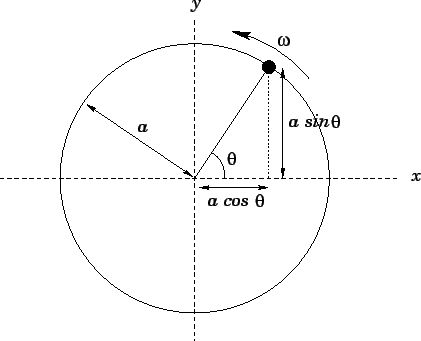Since the object is executing uniform circular motion, we expect the angleto increase linearly with time. In other words, we can write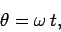(536)

whereis the angular rotation frequency (i.e., the number of radians through which the object rotates per second). Here, it is assumed that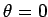at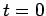, for the sake of convenience.

From simple trigonometry, the- and-coordinates of the object can be written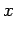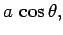(537)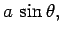(538)

respectively. Hence, combining the previous equations, we obtain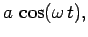(539)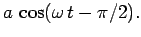(540)

Here, use has been made of the trigonometric identity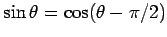. A comparison of the above two equations with the standard equation of simple harmonic motion, Eq. (505), reveals that our object is executing simple harmonic motion simultaneously along both the- and the-axes. Note, however, that these two motions are(i.e.,radians) out of phase. Moreover, the amplitude of the motion equals the radius of the circle. Clearly, there is a close relationship between simple harmonic motion and circular motion.Next: Worked example 11.1: Piston Up: Oscillatory motion Previous: The compound pendulum
Richard Fitzpatrick 2006-02-02